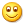44
int main(void) { uchar count = 0; uint i, j; NUM_1 = 0; //开启第一位数码管 car_stop(); //小车开始时停止 while(1) { IF (count > 9) //数码管显示计数值最大为9 { //计数值大于9后不再增加 count = 9; } P0 = table[count]; //数码管显示计数值 if (XJ_L == 0 && XJ_R == 0) //左右循迹输入都为白色 { //小车向前进 car_go(); } else if (XJ_L == 1 && XJ_R == 0) //左循迹输入为黑色，且右循迹输入为白色 { //小车向左转 car_turnleft(); } else if (XJ_L == 0 && XJ_R == 1) //左循迹输入为白色，且右循迹输入为黑色 { //小车向右转 car_turnright(); } else if (XJ_L == 1 && XJ_R == 1) //左右循迹输入都为黑色，即遇到计数标志 { BUZZ = 0; //开启蜂鸣器 for (i=0; i
2020-8-28 08:06:40评论淘帖 邀请回答

### 相关问题

4个回答
 你要实现一个什么功能呢？ 另外你的代码太乱了，最好一句换一行
 問題好像沒貼完整? 蜂鳴器改掉就只是把蜂鳴器控制的程式碼換成 car_stop(); 這樣子而已,可以再描述清楚一點 以上## 站长推荐/8•最新主题
•推荐主题
•热门主题
•我的帖子
-

-

-

-

-

-

-

-

-

-

-